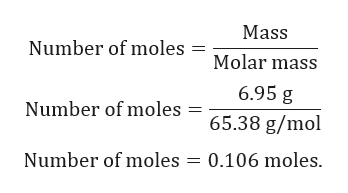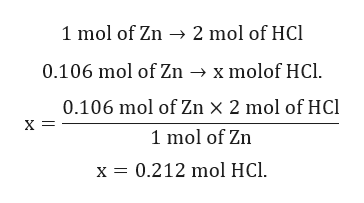# Zinc reacts with hydrochloric acid according to the reaction equation Zn(s)+2HCl(aq)⟶ZnCl2(aq)+H2(g) How many milliliters of 6.00 M HCl(aq) are required to react with 6.95 g Zn(s)? volume: _______ mL

Question
67 views

Zinc reacts with hydrochloric acid according to the reaction equation

Zn(s)+2HCl(aq)⟶ZnCl2(aq)+H2(g)

How many milliliters of 6.00 M HCl(aq) are required to react with 6.95 g Zn(s)?

volume: _______ mL
check_circle

star
star
star
star
star
1 Rating
Step 1

Given:

Mass of Zn = 6.95 g.

Molar mass of Zn = 65.38 g/mol.

Molarity of HCl = 6.0 M.

Balanced chemical reaction is given by:

Step 2

Calculation for number of moles of Zn:help_outlineImage TranscriptioncloseMass Number of moles = Molar mass 6.95 g Number of moles 65.38 g/mol Number of moles 0.106 moles. fullscreen
Step 3

Calculation of number of moles of HCl:

From the above balanced chemical reac...help_outlineImage Transcriptionclose1 mol of Zn 2 mol of HCl 0.106 mol of Zn -»x molof HCl 0.106 mol of Zn X 2 mol of HCl х- 1 mol of Zn 0.212 mol HCl x fullscreen

### Want to see the full answer?

See Solution

#### Want to see this answer and more?

Solutions are written by subject experts who are available 24/7. Questions are typically answered within 1 hour.*

See Solution
*Response times may vary by subject and question.
Tagged in

### Solutions